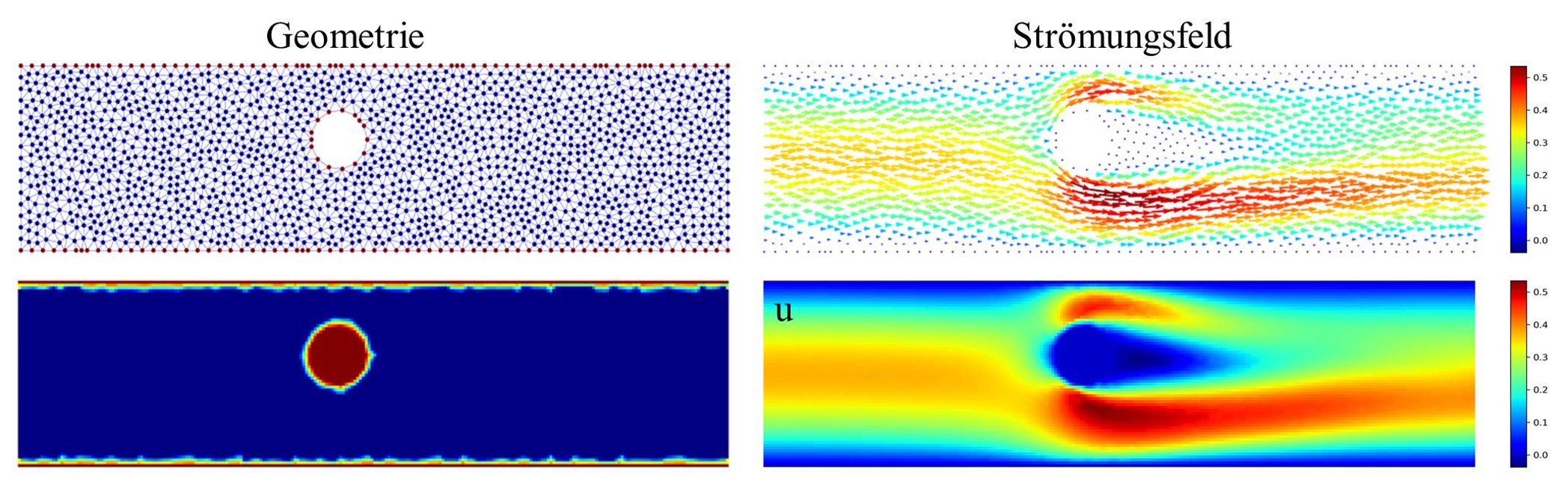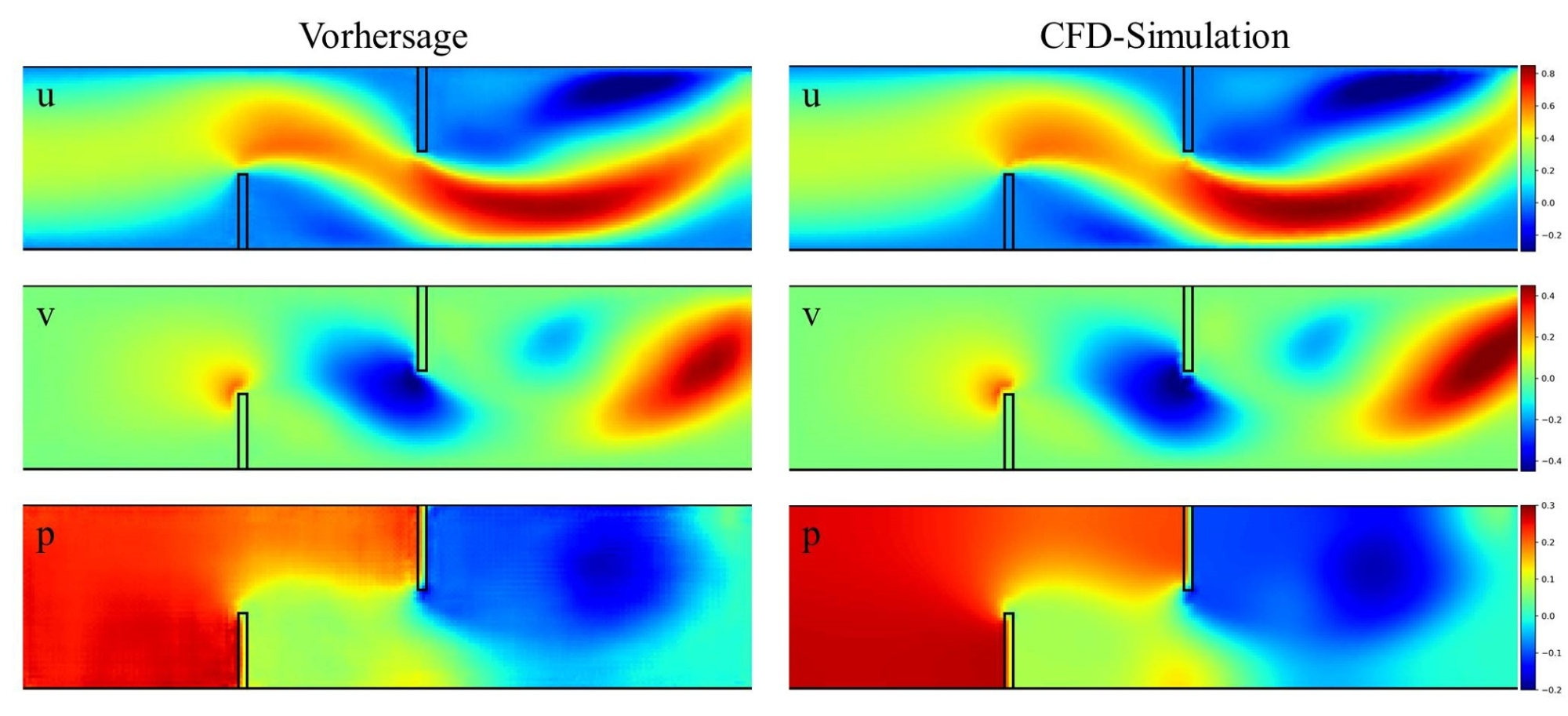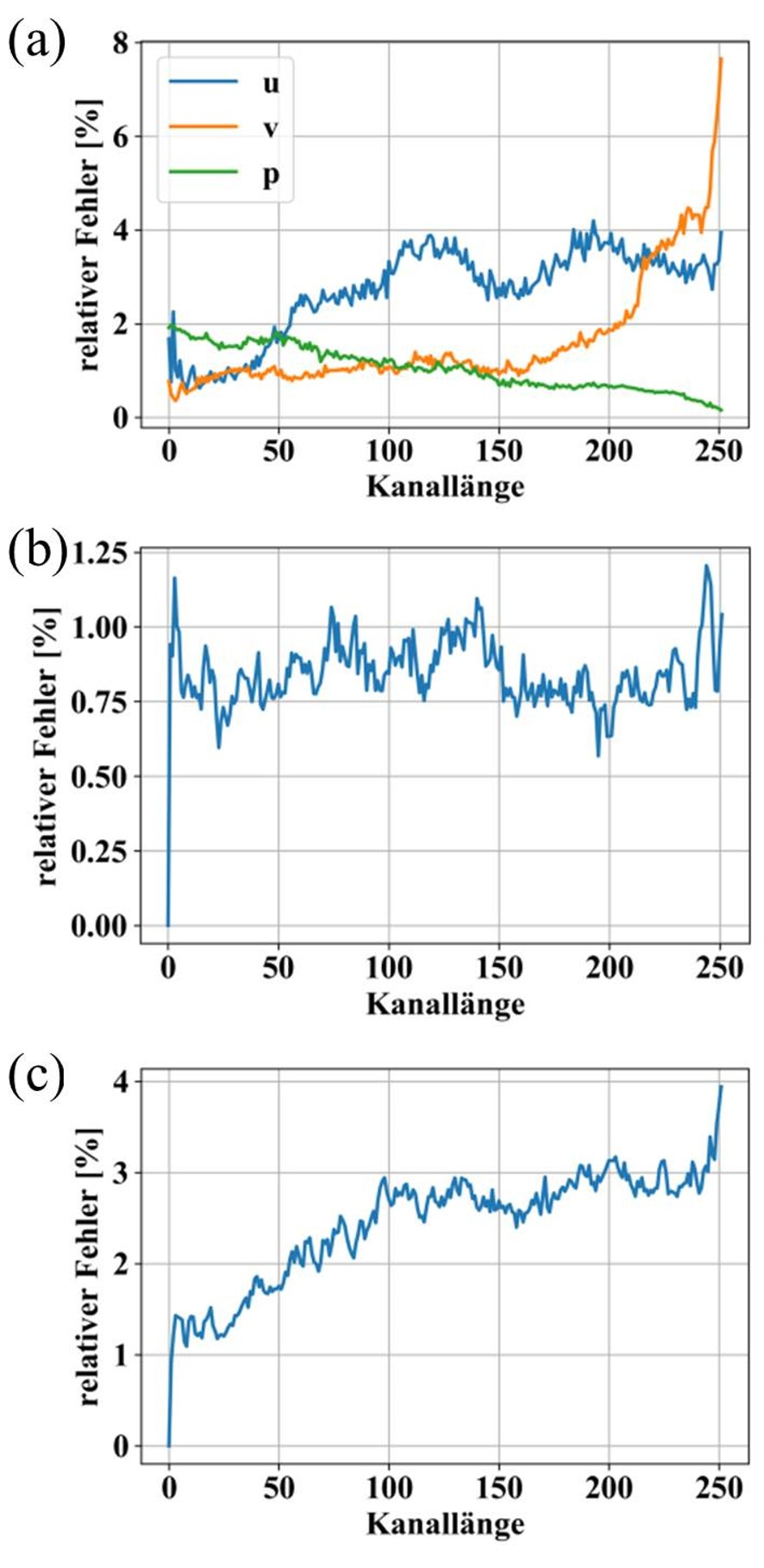# Prediction of Steady-State Flow Fields with Neural Networks

### Motivation

In a CFD simulation, physical problems are described by fluid mechanical equations and solved approximately with numerical methods. This procedure is generally computational intensive and time-consuming. Instead of solving a system of partial differential equations iteratively, the goal is to train a deep learning model that approximates the solution. Convolutional neural networks (CNNs) are suitable for this purpose. The CNN is to learn characteristics describing a flow in order to generate predictions for new cases. In this way, flow fields can be calculated much faster. However, it must be examined how high the quality loss compared to CFD simulations is.

### Dataset

The model used requires an extensive data set for the training process. Significant variation in the data is important in order to learn as many flow phenomena as possible. For this reason, a generic data set is created from CFD simulations. To keep the complexity low, the setup is limited to two-dimensional steady channel flows with different immersed geometries. CNN's cannot handle unstructured CFD grids. Therefore, the data must be interpolated to a Cartesian grid in a preprocessing step. This corresponds to an image, here with 64x256 pixels, where color-channels represent velocities (𝑢,𝑣) and pressure (𝑝). Then, the color-channels are normalized to the range of values [-1,1]. Following the same scheme, the geometry is transferred to an image, where all geometry-nodes represent one and the fluid-elements represent zero. The procedure is illustrated by an exemplary grid in Figure 1.Fig. 1: Interpolation of CFD simulation to images. Left: Computational grid with geometry and fluid elements. Right: The flow field, as well as the corresponding color-channel 𝑢.

### U-Net

The model for processing geometry information into flow fields is based on the U-Net architecture. This comprises two symmetric paths, shown in Figure 2. In the contraction path, the input data is compressed step by step. In this process, the network learns to extract the spatial information, e.g., position, arrangement, and size of the objects in the data. Then, the data is decompressed again in the expansion path and transformed into a flow field (𝑢,𝑣,𝑝). Cross-connections ensure that contextual information is available at each level of the expansion path.

### Results

To test the applicability of the trained model to new data (generalization), the U-Net is evaluated with 300 new CFD simulations. For each test sample, a flow field is predicted from the geometry. An example and the corresponding CFD simulation are shown in Figure 3.

For the evaluation of the generalization, the relative error between the predicted values and CFD simulation is calculated. The mean inflow velocity, as well as the given reference pressure, are used as reference variables. The U-Net achieves a relative error of 3.8%, 2%, and 1.1% for (𝑢,𝑣,𝑝), respectively. This shows that a relatively high precision of the individual flow variables can be achieved over the entire channel.Fig. 3: Example flow prediction for 𝑢, 𝑣, and 𝑝 (left), and the comparison values from CFD simulation (right).Fig. 4: Relative error over channel length: (a) 𝑢, 𝑣, and 𝑝 averaged over cross section, (b) conservation of mass, (c) conservation of energy.

Furthermore, the flow quantities, averaged over the cross-section are considered. Figure 4 (a) shows that the error in (𝑢,𝑣) increases over the channel length. This is because of the increasing variance in the downstream flow due to deflections. On the other hand, large pressure gradients due to stagnation points in front of the objects lead to a decreasing error in (𝑝).

Furthermore, the flow quantities averaged over the cross-section are considered. Figure 4 (a) shows that the error in (𝑢,𝑣) increases over the channel length. This is because of the increasing variance in the downstream flow due to deflections. On the other hand, large pressure gradients due to stagnation points in front of the objects lead to a decreasing error in (𝑝).

Finally, the deviation of the volume- and energy-flux over the length of the channel is examined. In each case, these are normalized to the incoming volume flow and energy flux, respectively. From Figure 4 (b), it can be seen that the deviation of volume flow is relatively constant between 0.8% - 1%. The errors in (𝑢) largely cancel each other out, resulting in a small effect on mass conservation. In contrast, Figure 4 (c) shows an increasing deviation in energy conservation from 1.2% to 4%. Here, the errors in (𝑢,𝑣) visibly affect the conservation of energy.